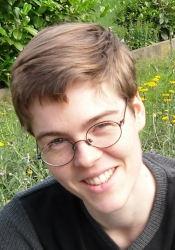## Lilian Matthiesen

Associate professorKTH
Department of Mathematics
Lindstedtsvägen 25
100 44 Stockholm
Sweden

My research interests lie in the area of combinatorial and analytic number theory.

### Preprints and papers

1. Frobenian multiplicative functions and rational points in fibrations (with D. Loughran). Submitted. arXiv.
2. Linear correlations of multiplicative functions. Proc. London Math. Soc., to appear. arXiv.
3. A consequence of the factorisation theorem for polynomial orbits on nilmanifolds. (Corrigendum to Acta Arith. 154 (2012), 235-306.) arXiv pdf
4. On the square-free representation function of a norm form and nilsequences. J. Inst. Math. Jussieu 17 (2018), 107-135. arXiv
5. Generalized Fourier coefficients of multiplicative functions. Algebra Number Theory 12 (2018), 1311-1400. arXiv
6. Norm forms for arbitrary number fields as products of linear polynomials (with T. Browning). Ann. Sci. Ecole Norm. Sup. 50 (2017), 1375-1438. arXiv
7. Rational points on pencils of conics and quadrics with many degenerate fibres (with T. Browning and A. Skorobogatov). Annals of Math. 180 (2014), 381-402. arXiv
8. Correlations of representation functions of binary quadratic forms, Acta Arith. 158 (2013), 245-252. arXiv
9. Linear correlations amongst numbers represented by positive definite binary quadratic forms, Acta Arith. 154 (2012), 235-306. arXiv
10. Correlations of the divisor function, Proc. London Math. Soc. 104 (2012), 827-858. arXiv*
11. There are uncountably many topological types of locally finite trees, J. Comb. Theory, Ser. B 96 (2006), 758-760. pdf
*differs from the published version.